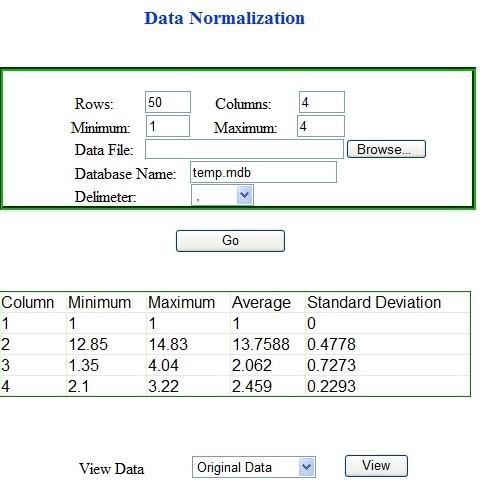Home

# Data Normalization using ASP.NET

## Problem Statement

In any datasets each attribute has its own range. For instance an age attribute can have a maximum range up several hundred. On the contrary, monthly salary attribute will always have range in thousands. Abnormal minimum and maximum values (noise) can also lead to misinterpretation of data.

Data Normalization uses different techniques to narrow down values to a certain range. Min-Max and z-Score are most common normalization techniques.

In this tutorial we will use ASP.NET to solve the data normalization problem. A web-based application will a read dataset, normalize it, and store it in a Microsoft Access database. The normalized dataset will be displayed to the user in an ASP.NET data grid control.

For the sake of convenience we will divide the problem solving process into these phases:

• Finding out the statistics (Average, Minimum, Maximum and Standard Deviation)

• Normalize and store data

• Display data

First of all start your Microsoft Visual Studio. To build an ASP.NET web application go to File menu and select New>Website (Note that you can skip this part and download complete project, used in this tutorial). In the new dialogue box specify path and select Visual C# as language. Now place the following controls from toolbar to your Default.aspx (while in the design view):

• Five text Boxes (For rows, columns, minimum, maximum and database name). You can set the Text properties for the text boxes in the properties window (just select control, press F4 and edit the "Text" option)

• One DropDownList

• One Button control for starting the execution of code.

• You can also place a Label if you want to set an heading for the application

Place all controls inside a Panel control and name the controls for your convenience. Now your ASP.NET application should look something like this: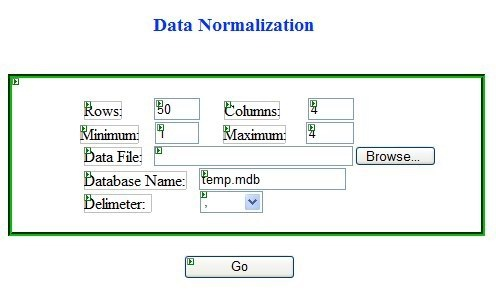Now double click the Go button to start the coding. Here is the ASP.NET code for reading and parsing the input file:

// create an object of StreamReader and pass file path as parameter

string> line;
string>[] tokens;

// save delimeter specified  by user
string> temp = DelmDropDown.Text;

// convert from string to character
char>[] delimeters = { Convert.ToChar(temp) };

// read till end of file or till specified rows
while> (!sr.EndOfStream && i < rows)
{

// split the line string into array of characters on basis of delimeter
tokens = line.Split(delimeters);

for> (int j = 0; j < columns; j++)
{
// save each value to our original matrix "OrigMatrix"
OrigMatrix[i, j] = Convert.ToDouble(tokens[j]);
}
++i;
}

For file parsing in ASP.NET, we used StreamReader class object. To use the class you first have to include System.IO namespace at the top of Default.aspx.cs file. OrigMatrix is a 2-D matrix for storing the parsed data. Size of matrix will be specified by user in the rows and column text area. Convert.ToDouble() function is for string to double conversion. At the end of this function all our required data will be stored in OrigMatrix.

After doing the calculation we want the ASP.NET application to save values to a MS Access database. First of we have to create an Access database having five attributes, namely Column_1, Column_2, Column_3, Column_4 and Type. Data type of the "Type" attribute is Text, whereas rest attributes are of type Double Number. Name the table NormalizedData (you can also give any other name). Save the database file in C:\Inetpub\wwwroot. If you have installed Windows on some other drive than change the path accordingly.

In ASP.NET we use the connection object to connect to a database. You have to include the name space System.Data.OleDb for this purpose. The code for establishing connection is as follows:

OleDbConnection conn = new OleDbConnection();  // our connection object
string DbPath = @"Provider=Microsoft.Jet.OLEDB.4.0;"// data provider
@"Data Source=C:\inetpub\wwwroot\"+ DbTextBox.Text; // database path
conn.ConnectionString = DbPath;
conn.Open();    // open the connection

For executing a command in ASP.NET we need to declare an initialize a new object belonging to the OleDbConnection class. Here is the code for initializing the command object:

OleDbCommand command = new OleDbCommand();
command.Connection = conn;     // conn that we have already initialized
command.CommandText = MyCmd;   // â€œMyCmdâ€ is string containing actual command
command.CommandType = CommandType.Text;
command.ExecuteNonQuery();

You can also place the above code inside a function so that it can be reused when required.

public void ExecuteCommand(string MyCmd)
{
// our command execution code goes here
}

For storing data to the database we will construct a query by executing a nested loop that traverses the whole matrix. Following code snippet accomplishes this task:

string ValuesStr="";
string CmdStr = "";

for (int i = 0; i < rows; i++)
{
for (int j = 0; j < columns; j++)
{
ValuesStr += OrigMatrix[i, j] + ",";
}
// insert the row/record in database here
CmdStr = "INSERT INTO NormalizedData VALUES(" +
ValuesStr + "'original')";

ExecuteCommand(CmdStr);  // use our function to execute command
ValuesStr = "";
}

## Finding out the statistics

In ASP.NET doing calculations is very easy. We will write a function to perform these calculations. Each column will have its own statistics. We will store each statistic in a separate 1-D array. Here is the C Sharp code that for performing the desired task:

for (j = 0; j < columns; j++)
{
// initialize values at start of each column loop
// always consider first element in each column as Max and Min
Min = OrigMatrix[0, j];
Max = OrigMatrix[0, j];

Sum = 0;

for (i = 0; i < rows; i++)
{
if (OrigMatrix[i, j] > Max)
Max = OrigMatrix[i, j];

if (OrigMatrix[i, j] < Min)
Min = OrigMatrix[i, j];

Sum += OrigMatrix[i, j];
}

MinArray[j] = Min;
MaxArray[j] = Max;
AverageArray[j] = (Sum / rows);
}

// calculate standard deviation

// calculate difference from mean, square it and sum it
for (j = 0; j < columns; j++)
{
Sum = 0;
Avg = 0;

for (i = 0; i < rows; i++)
{
Sum += Math.Pow((OrigMatrix[i, j] - AverageArray[j]), 2);
}

// varience
Avg = (Sum / rows);

// standard deviation is squre root of varience
StdArray[j] = Math.Sqrt(Avg);
}

Min, Max, Sum and Avg are variables of double type and i and j are integer loop counters. MinArray, MaxArray[j], StdArray[j] are our arrays of double type for for saving minimum, maximum and standard deviation of each column.

## Normalize and store data

After doing the basic calculation we will proceed towards the normalization phase. Here is the ASP.NET code for finding and storing the z-Score and Min-Max:

double MinMaxValue = 0;
string MinMaxStr = "";

double zValue = 0;
string zStr = "";

for (int i = 0; i < rows; i++)
{
for (int j = 0; j < columns; j++)
{
// Apply Min-Max Normalization
MinMaxValue = ReturnMinMax(OrigMatrix[i, j], j);
MinMaxStr += MinMaxValue + ",";

// Apply z-Score Normalization
zValue = ReturnZScore(OrigMatrix[i, j], j);
zStr += zValue + ",";
}

// insert the row/record in database here
string CommandStr = "INSERT INTO NormalizedData VALUES(" +
MinMaxStr + "'min-max')";

// call the command execution function
ExecuteCommand(CommandStr);

// insert the row/record in database here
CommandStr = "INSERT INTO NormalizedData VALUES(" +
zStr + "'z-score')";

ExecuteCommand(CommandStr);

MinMaxStr = "";
zStr = "";
}

ReturnMinMax and ReturnZScore are our own function. They take value and column no as parameter and return the results.

## Display data

In ASP.NET, easiest way to display contents of a database is by using a Data Grid. First we need to create a separate web form. Go to File menu and select New>File option. Specify name and language (Visual C#). Place a GridView control (present in the Data section) from the Toolbox.

In our previous form we will place some useful values in the Session object. Place a DropDownList in the default form. While in the aspx file, select the DropDownList. A small arrow will apperar at its top right corner. Clicking the arrow reveals a menu for DropDownList Tasks.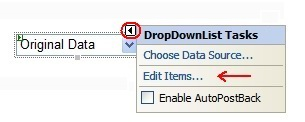Select the link named "Edit Items...". Add items to the DropDownList by clicking the Add button and specifying Text and Value attributes for each item.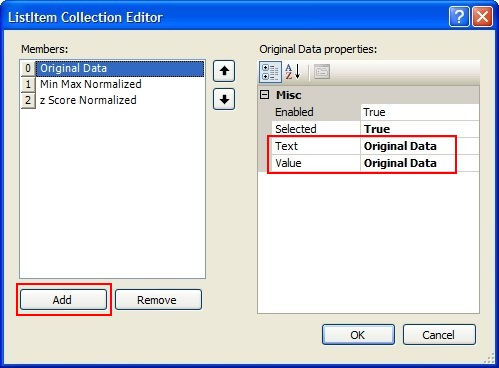Now place a button for viewing data. Write the following code in its click handler:

// place values in session according to selected option
switch (option)
{
case 0:
Session["type"] = "original";
Session["title"] = "Orininal Data View";
break;

case 1:
Session["type"] = "min-max";
Session["title"] = "Data after Min-Max Normalization";
break;

case 2:
Session["type"] = "z-score";
Session["title"] = "Data after z-Score Normalization";
break;
}

// move to the page DataView.aspx, one with the data grid
Server.Transfer("DataView.aspx");

In the newly created ASP .NET web form's C Sharp file (Dataview.asp.cs) retrieve values from the session object and display them on the data grid. In the Page_Load event just write the following code:

//get type from session. original,min-max or z-score
string TypeStr = Convert.ToString( Session["type"] );

//also get table path from session
string DbPath = Convert.ToString( Session["path"] );

TitleLabel.Text = Convert.ToString(Session["title"]);
// get title from session

string MyQueryStr = "SELECT Column_1, Column_2, Column_3, Column_4"+
" from NormalizedData where Type='" + TypeStr + "'";

OleDbConnection conn = new OleDbConnection(DbPath);
DataSet ds = new DataSet();  // create an new object of DataSet class

da.Fill(ds, "MyDataSet");
MyGrid.DataSource = ds;
MyGrid.DataBind();

Above code can be used for all three type of data namely original, min-max and z-score. Depending upon the "type" stored in the session. Don't forget to include t System.Data.OleDb namespace at top of the file.

In ASP.NET data adapter object "da" acts like a bridge. It establishes connection between our DataSet object "ds" and the MS Access database "temp.mdb". Data adapter can not only read records from a database, but it can also delete records, edit records and store new records.

ASP .NET DataSet object "ds" is a disconnected array. It acts like a cache. When you fill dataset using the data adapter object, values are stored to the data set object. Later on these values can be retrieved without connecting to database again.

Here is a screenshot of the application showing normalized data: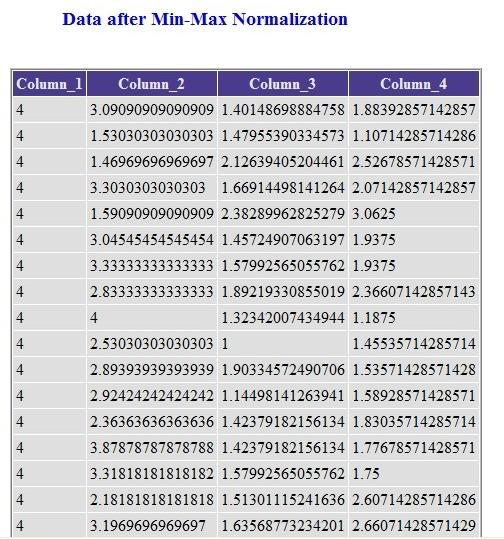We can also use a Table control to show the statistics in Default.aspx file. For this purpose we will have to use objects of TableRow and TableCell classes. Here is an example.

int numrows = 5;    // no of rows in the table
int numcells = 5;   // no of cells in each row

for (int j = 0; j < numrows; j++)
{
TableRow r = new TableRow();    // create a new row object
for (int i = 0; i < numcells; i++)
// each row will have cells equal to "numcells"
{
TableCell c = new TableCell();  // create object for cell c.Controls.Add(new LiteralControl("Column")); //set text for cell